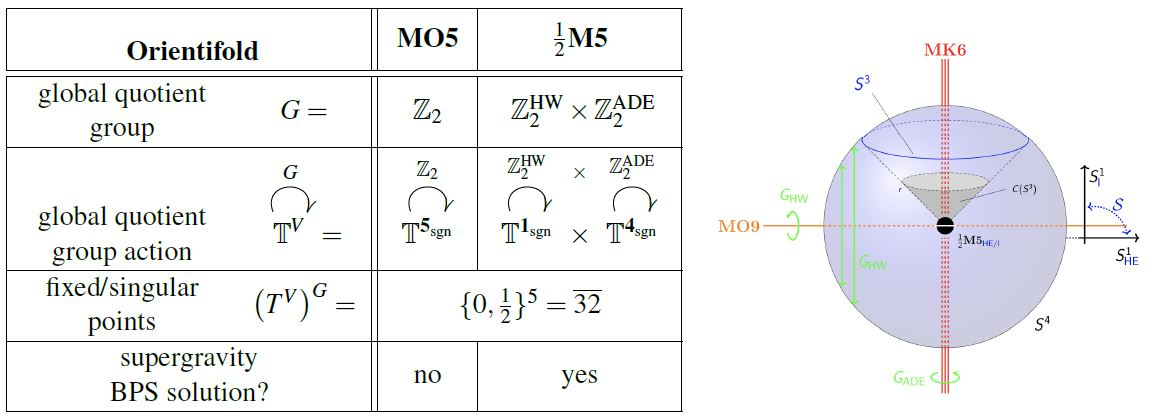Contents

# Contents

## Idea

The MO5 (Dasgupta-Mukhil 95, Sec. 2FFurther, Witten 95, Sec. 3.3) is one of the types of $\tfrac{1}{2}$ BPS orientifold fixed loci in M-theory/on D=11 N=1 super-spacetime. Locally on super Minkowski spacetime it is the super-orientifold $\mathbb{R}^{10,1\vert \mathbf{32}} \sslash \mathbb{Z}^{\Gamma^{56789}}_2$ given by the global orbifold quotient by the group of order 2 which is generated by the Clifford algebra-element $\Gamma_5 \Gamma_6 \Gamma_7 \Gamma_8 \Gamma_9 \in Pin^+(10,1)$ in the positive pin group (for precise details see HSS 18, Lemma 4.12).

## Properties

### Charge, anomaly cancellation and duality to O4-planes

Under duality between M-theory and type IIA string theory, for KK-compactification along a circle-fiber parallel to the MO5, a plain MO5 becomes the O4-plane, specifically the $O^- 4$-plane, while an MO5 with an M5-brane on top of it becomes the $O^+ 4$-plane (Gimon 9, Sec. III8,Hanany-Kol 00, Sec. 3.1).

Hence the spacetime dimensions of the MO5 is the same that of the black M5-brane, but its charge as an O-plane is $- \tfrac{1}{2}$ that of a single M5-brane (Dasgupta-Mukhil 95, Sec. 2, Witten 95, 3.3, Hori 98, 2.1), so that am M-theoretic lift of RR-field tadpole cancellation requires the presence of 16 M5-branes in an toroidal MO5-type orientifold $\mathbb{T}^{\mathbf{5}_{sgn}} \!\sslash\! \mathbb{Z}_2$.

### As a sector of heterotic M-theory on ADE-singularities

By the classification of MFF 12, Sec. 8.3, the MO5-plane is not in fact a $\tfrac{1}{2}$ BPS solution of D=11 N=1 supergravity. But the intersection of an MK6 with an MO9-plane is a $\tfrac{1}{4}$ BPS solution to D=11 N=1 supergravity.graphics grabbed from HSS18, Example 2.2.7

Thus, heterotic M-theory on ADE-orbifolds the MO5-plane becomes the fixed locus of the diagonal $\mathbb{Z}_2 \overset{ diag }{\hookrightarrow } \mathbb{Z}_2 \times G^{DE}$, hence the intersection of an MO9 with an MK6${}_{DE}$ (FLO 99, Sec. 4), also called the $-\tfrac{1}{2}M5$, since under duality between M-theory and type IIA string theory this becomes the half NS5-brane of type I' string theory.

### As geometric engineering of $D=6$$\mathcal{N} = (2,0)$ SCFTs

Coincident M5-branes on an MO5-plane are supposed to geometrically engineer D=6 N=(2,0) SCFTs with D-series gauge groups (AKY 98, Sec. IIB).

But if these are really $-\tfrac{1}{2} M5 = MK6 \cap MO9$-branes of heterotic M-theory on ADE-orbifolds, then they will geometrically engineer D=6 N=(1,0) SCFTs, instead, see there.

### General

The original articles are

Classification view of rational equivariant Cohomotopy of super-spacetime:

### As a sector of the $-\tfrac{1}{2}M5$ in heterotic M-theory at ADE-singularities

Discussion in the more general context of heterotic M-theory on ADE-orbifolds is in

and in view of super-embeddings of M5-branes in

### Relation to O4-planes

Relation of the MO5 to the O4-plane under duality between M-theory and type IIA string theory:

### Geometric engineering of orthogonal $D=6$, $\mathcal{N}=(2,0)$ SCFTs

• Changhyun Ahn, Hoil Kim, Hyun Seok Yang, $SO(2N)$ $(0,2)$ SCFT and M Theory on $AdS_7 \times \mathbb{R}P^4$, Phys.Rev. D59 (1999) 106002 (arXiv:hep-th/9808182)